Question

# what is the discount period, bank discount, and proceeds on 25000.00 at 9% for 60 days, date of note is June 8 and date note discounted is July 10

what is the discount period, bank discount, and proceeds on 25000.00 at 9% for 60 days, date of note is June 8 and date note discounted is July 10?

We need at least 10 more requests to produce the answer.

0 / 10 have requested this problem solution

The more requests, the faster the answer.

All students who have requested the answer will be notified once they are available.

#### Earn Coins

Coins can be redeemed for fabulous gifts.

Similar Homework Help Questions
• ### Use ordinary interest: Principal \$70,000 Rate of Interest: 11% Time: 90 days Maturity Value: A Date note made Mar 10 Date note discounted: April 15 discount period: B proceeds: C note to be discounted at 10%

Use ordinary interest: Principal \$70,000 Rate of Interest: 11% Time: 90 days Maturity Value: A Date note made Mar 10 Date note discounted: April 15 discount period: B proceeds: C note to be discounted at 10%

• ### Date of Note March 11, length of note 200 days, Date of discount, June 28

Date of Note March 11, length of note 200 days, Date of discount, June 28. What is the maturity date and the discount period, no leap years, exact days

• ### 8 On October 29, 2019, the Berkeley Company accepted a 60-day, 9 percent note from Devon...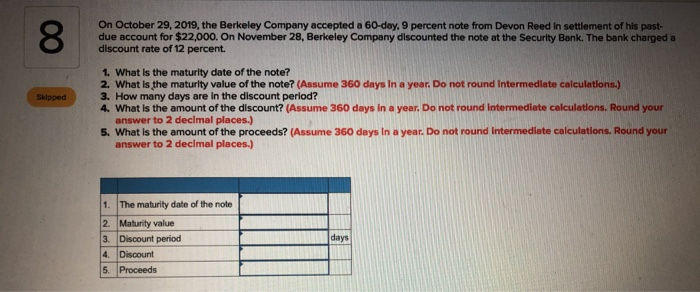8 On October 29, 2019, the Berkeley Company accepted a 60-day, 9 percent note from Devon Reed in settlement of his past- due account for \$22,000. On November 28, Berkeley Company discounted the note at the Security Bank. The bank charged a discount rate of 12 percent. 1. What is the maturity date of the note? 2. What is the maturity value of the note? (Assume 360 days in a year. Do not round Intermediate calculations.) 3. How many days...

• ### The proceeds of a \$7,500.00, 10% simple discounted note for 85 days is:

The proceeds of a \$7,500.00, 10% simple discounted note for 85 days is:

• ### Solve for maturity value, discount period, bank discount, and proceeds.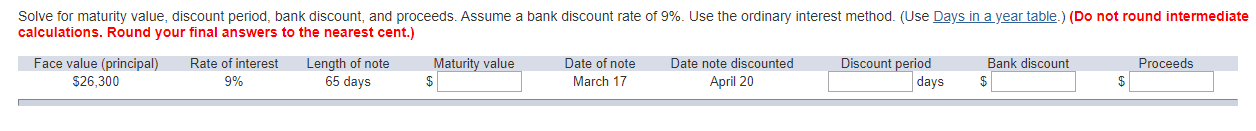Solve for maturity value, discount period, bank discount, and proceeds. Assume a bank discount rate of 9%. Use the ordinary interest method. (Use Days in a year table.) (Do not round intermediate calculations. Round your final answers to the nearest cent.)

• ### Anderson Co. issued a \$55,649, 60-day, discounted note to National Bank. The discount rate is 8%....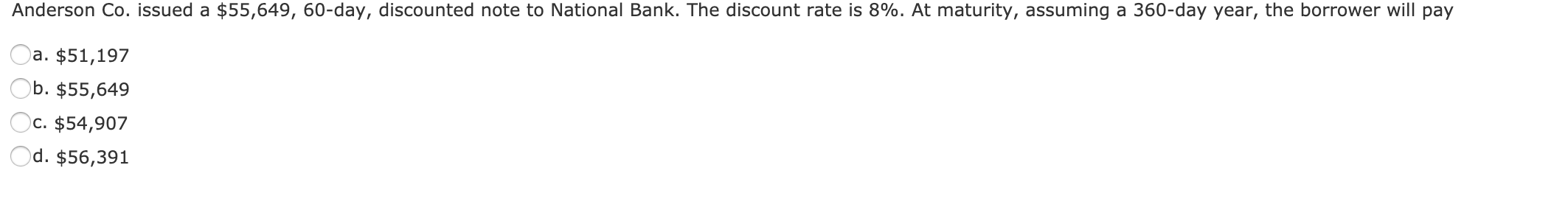Anderson Co. issued a \$55,649, 60-day, discounted note to National Bank. The discount rate is 8%. At maturity, assuming a 360-day year, the borrower will pay a. \$51,197 Ob. \$55,649 Oc. \$54,907 Od. \$56,391

• ### Actuarial Science Mathematics of Finance Discounted Value 6. For cach promissory note in the following table, determine the maturity date, maturity value, dis- counted period, and the proceeds. No.Da...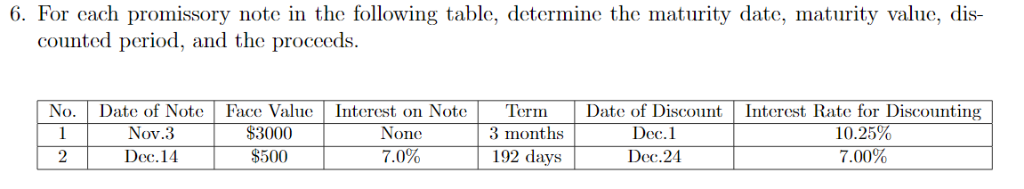Actuarial Science Mathematics of Finance Discounted Value 6. For cach promissory note in the following table, determine the maturity date, maturity value, dis- counted period, and the proceeds. No.Date of Note Face ValueInterest on NoteTermDate of Discount Interest Rate for Discounting Nov.3 \$3000 None Dec.1 3 months \$500 Dec.24 192 days 7.00% 2 Dec.14 7.0% 6. For cach promissory note in the following table, determine the maturity date, maturity value, dis- counted period, and the proceeds. No.Date of Note Face...

• ### Bill Blank signed an \$7,540 note at Citizen's Bank. Citizen's charges a 8.2% discount rate. Assume...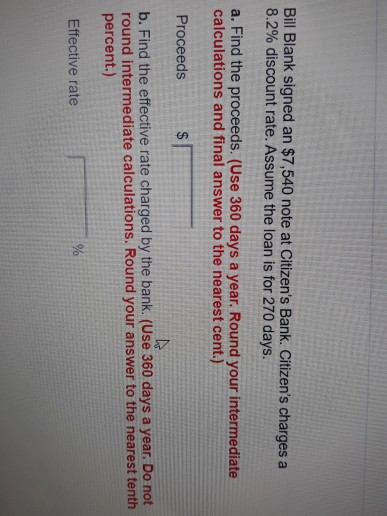Bill Blank signed an \$7,540 note at Citizen's Bank. Citizen's charges a 8.2% discount rate. Assume the loan is for 270 days. a. Find the proceeds. (Use 360 days a year. Round your intermediate calculations and final answer to the nearest cent.) Proceeds b. Find the effective rate charged by the bank. (Use 360 days a year. Do not round intermediate calculations. Round your answer to the nearest tenth percent.) Effective rate You were offered the opportunity to purchase either...

• ### A 60-day, 9% discounted note for \$26,400 is received from a customer on account on May 1. What are the proceeds from the...

A 60-day, 9% discounted note for \$26,400 is received from a customer on account on May 1. What are the proceeds from the note on May 1? Select the correct answer. \$396 \$26,400 \$26,004 \$26,796

• ### On May 20, Sheffield Company discounted at Sunshine Bank a \$7,290 (maturity value), 139-day note ...

On May 20, Sheffield Company discounted at Sunshine Bank a \$7,290 (maturity value), 139-day note dated Feb. 20. Sunshine’s discount rate was 8%. (Use Days in a year table.) What proceeds did Sheffield Company receive? (Use 360 days a year. Do not round intermediate calculations.) Proceeds received :?